$\require{cancel}$

# Some Other Components

• We are going to look at some of the things that are standard components.
• I will get just a little out of order and present some sequential logic as well. (I want a register).
• In the end, we will build the shell of a Simple CPU.
•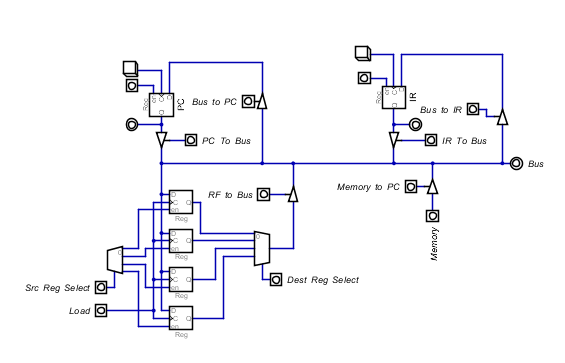• This uses for three modules
• A register
• A Multiplexor/Demultiplexor
• An Encoder/Decoder
• The register
• This is way out of order so we will only use the unit here.
• We will build one later.
• This is a unit of memory.
• Probably word size.
• It has two data lines
• The line marked D is an input data line.
• The line marked Q is an output data line.
• Q constantly (sort of) supplies the data stored in the register.
• There are delays when the register is changing when the data might not be correct.
• There are at two control lines in this register.
• The en line makes the register active, ie respond to change.
• The C line represents the clock.
• By default, the register has some value.
• To change the value
• Put data on the D line (and have it stabilize)
• Raise enable from low to high.
• Raise the clock from low to high.
• Hold enable and D for the required time.
• Lower enable.
• This is called an edge triggered device.
•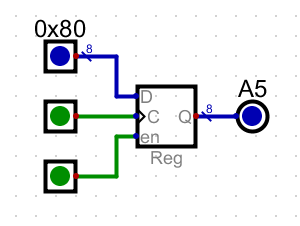• De/Encoders
• A decoder
• n selector bits as input.
• $2^n$ output bits.
• The value of the input is reflected in the output.
•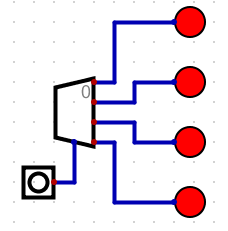• This is relatively easy to build.
•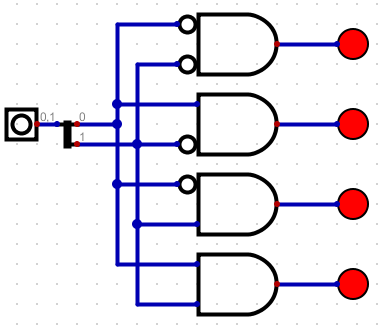• An encoder
• Has n output bits
• $2^n$ input bits.
• But only one of these lines can be lit at a time.
• The output represents the input line that was lit.
• The circuit "encodes" the address of the line that is lit.
•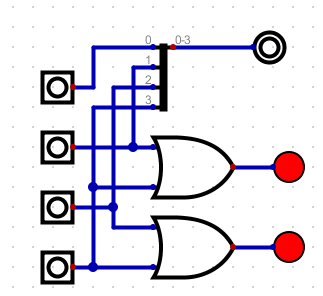• Multiplexor (mux) and demultiplexor
• A mux takes
• $2^n$ input data lines
• $1$ output data line.
• 1 control line with $n$ bits.
• Based on the value of the control line, the data from the selected input line is transferred to the output line.
•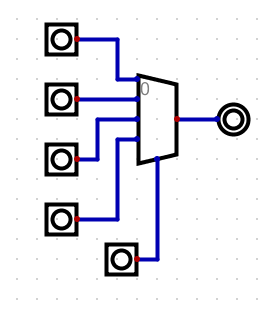• And is not that hard to build
•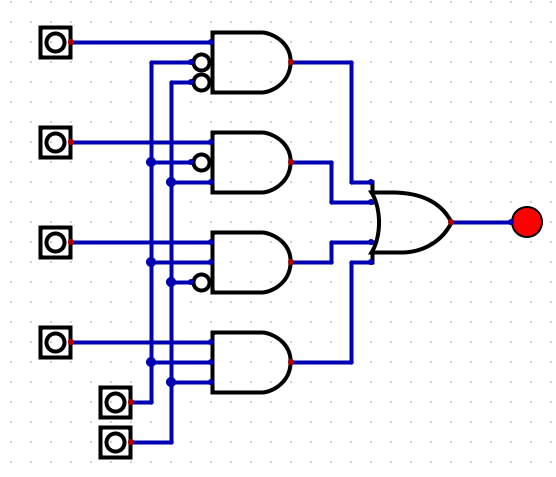• Figure B.3.6 on page 709 shows the implementation of a multi-channel MUX.
• A demultiplexor does the opposite.
• One data input line.
• $2^n$ data output lines.
• $n$ bit control line.
• Sends the output to the data line selected by the control line.
•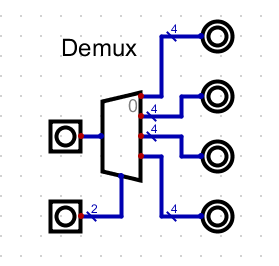• All of these circuits are available in parts.dig